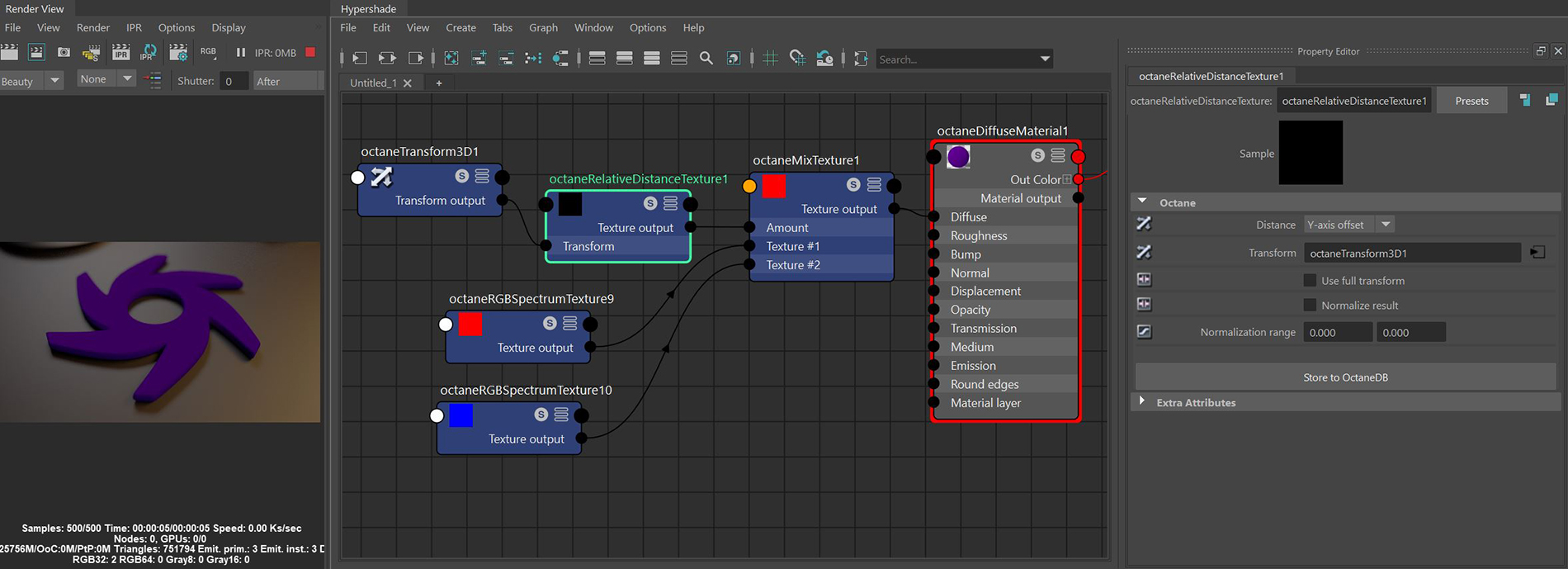# Relative Distance Texture

The Relative Distance node converts the distance to the origin from one of the specified reference transformations into a greyscale texture. Figure 1 is a simple example of using the Octane 3D Transform node offset in the Y direction as the mix amount for an Octane Mix Texture node.Figure 1: Using the Relative Distance node as a mix amount for red and blue RGB Spectrum nodes

### Relative Distance Texture Attributes

Distance Mode - Returns the Euclidean distance between the shaded point and the origin of the specified reference transform.

Reference Transform - Transform defining the coordinate system used to compute the distance metric.

Use Full Transform - If Enabled, the distances will be calculated after applying the full reference transform, including scale and rotation. If Disabled, only the translation is taken into account.

Normalize Results - Takes the result and normalizes the values between 0 and 1.

Normalization Range - Defines the minimum and maximum of the normalization range.